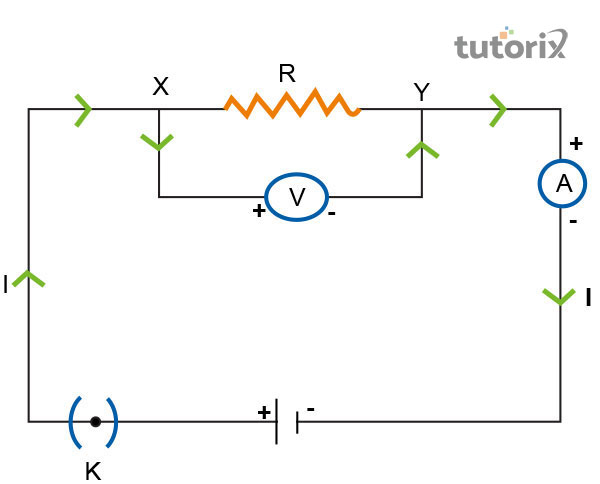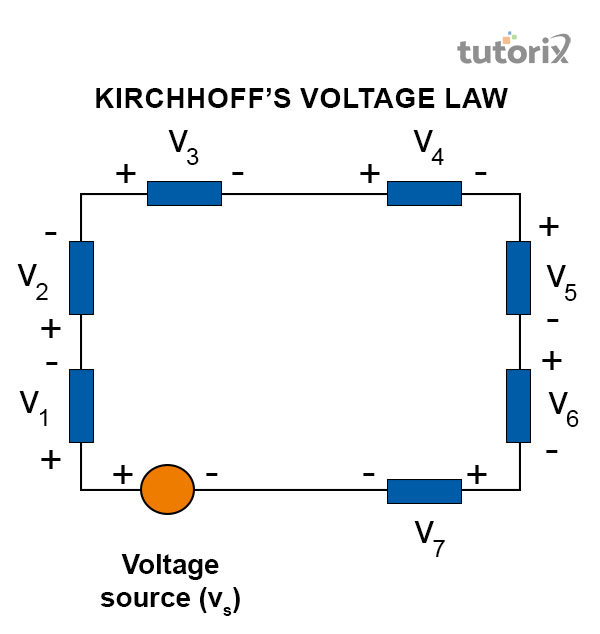# The Heating Effect of Current

## Introduction

The process of the heating effect of electric is attached to the daily human lives as most of the components used in daily activities are influenced by this phenomenon. Daily components like toaster, electric iron, heater and electric kettle are deeply moved by this process which makes it a significant part of the daily human lives. When a flow of current passes through a conductor it generates head as the hindrance is higher within the conductor this process influences the heating effect of eclectic in real-time.

### Discussion on the heating effect of current

The heating effect of current is a widely known phenomenon that happens in almost every part of daily human life. When the electrical appliances are used in real-time, the chemical reactions within the cells sets the electrons in motion. Electrons are present in the molecular structure of almost every electrical substance. Therefore, when the electrons are set on a certain motion, it generates hindrance within the conductors, eventually instigating the process of heating within the conductor.Figure 1: Heating effect of the current

The source of current needs a specific amount of energy to maintain its flow. The remaining energy generated in the process is expanded or exploited in the form of heat, especially when the temperature increases within the electrical appliances (Fang, 2020). When an electrical circuit is used, the energy within the circuit stands purely resistive and it is dissipated' in the form of energy in real-time. This eventually causes heating of current within the electrical appliances in real-time.

### Kirchhoff’s Law

Kirchhoff's law is an effective law in terms of quantifying the way current flows through a circuit and the way voltage varies within an electric circuit on a loop. Kirchhoff's law is segmented into two different parts, one is the current law of Kirchhoff and the other one is the voltage law of Kirchhoff. Kirchhoff’s law uses the Maxwell's equation to prove how the law can be obtained in real-time

### Kirchhoff’s current law

According to the current law of Kirchhoff, current can be defined as the rate of charge that is passing through a conducting medium. Based on that, the law states that the entering node and the leaving node of the total current are exactly equal to each other (Geeksforgeeks, 2022). In simple words, the current that is entering a junction through an electrical medium is exactly equal to the current that is leaving the junction at the same time .

This law by Kirchhoff is regarded as the first law of the heat effect of the current that deals with the conversion of charge when current flows through a certain medium. According to the formula, the total of the currents is 0 when the current is entering a node (Sciencefacts, 2022). The law also states that when a current enters a junction it acts as positive but when it leaves the junction it becomes negative..

### Kirchhoff’s voltage law

The voltage law of Kirchhoff depends on the electrical differences within the DC circuit when the closed-loop stands at zero. According to this law, the potential of electric represents the concentration of energy within a circuit. Immediately after the potential is released, it starts to spread to the uniform value, especially in the uninterrupted sections of the electrical circuits (Cui et6 al. 2018). This difference is termed voltage according to the principles of the heating effect of electricFigure 2: Kirchhoff’s voltage law

The voltage law by Kirchhoff states that within a closed-loop network the sum of voltage drops when the value of the lop stands equal to 0. This law by Kirchhoff is widely accepted by the name of conservation of energy as it deals with energy and voltage at the same time (Sciencefacts, 2022). The law also stresses the fact that when an electric node enters or leaves an electrical circuit a closed-circuit path is formed that eventually increases the heat effect within the electrical circuits in real-time.

### Applications of the heating effect of current

The heating effect of current has a widespread application in the daily human lives as it is applied in most electrical appliances in real-time. It is used in the electric iron where the mica is placed as an insulator by nature. In addition, it is used the electric bulbs that contain a highly resistive tungsten metal. It also consists of thick metallic wires which eventually makes it dependable in the phenomenon of the heating effect of electric. Additionally, it also has an application on other appliances like eclectic fuse, electric heater, ‘electric power and many other electrical devices.

## Conclusion

In the phenomenon of the heating effect of the current when a flow of current pass through a conductor, the temperature of the conductor increases due to the hindrance of the flowing current it eventually causes the heat effect of the flowing current in real-time. The main process is finished when the hindrance of the current flow generates heat within a conductor.

### FAQs

Q1. Which factors are crucial for maintaining the heating effect of the current?

The process of heating effect in an electrical component depends on three significant factors, the resistance, time of current flow and R of the conductor. The three factors are crucial for maintaining the heating effect of current in real-time.

Q2. Which electrical component can prevent the heating effect of current in real-time?

Fuse is the only electrical component that can prevent the heating effect while functioning as a medium for current flow. It also helps in preventing damages caused by the heating effect of current.

Q3. What are the formulas of Kirchhoff’s Current law and Voltage law?

The formula of Kirchhoff's first law (Current law) is $\mathrm{\sum\:Iin\:= \sum \:Iout\:}$. The formula for the second law (Voltage law) is $\mathrm{\sum \:Vtotal\: =\: 0}$.

Updated on: 18-Aug-2023

422 Views